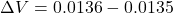Question

The coefficient of linear expansion of copper is 17 × 10-6 K-1. A block of copper 30 cm wide, 45 cm long, and 10 cm thick is heated from 0°C to 100°C What is the change in the volume of the block?

1.The change in volume is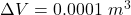Explanation:

From the question we are told that

The coefficient of linear expansion is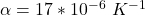The width  of the block is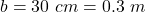The length is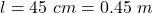The thickness is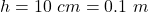The initial temperature is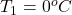The final temperature is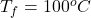The  initial volume is  mathematically represented assubstituting values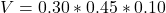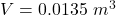Generally the expansion equation is mathematically represented as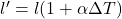where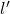is the new length

substituting values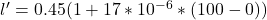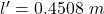The new width is evaluated as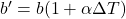substituting values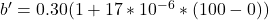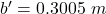The new thickness is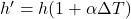substituting values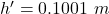The new volume is  mathematically evaluated as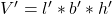substituting values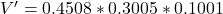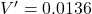Therefore